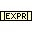# Expression Node function

Object information
Owning palette(s) Numeric palette
Type Function
Requires Basic Development Environment
IconUse the Expression Node function to calculate expressions that contain a single variable. The following built-in functions are allowed in formulas: `abs, acos, acosh, asin, asinh, atan, atanh, ceil, cos, cosh, cot, csc, exp, expm1, floor, getexp, getman, int, intrz, ln, lnp1, log, log2, max, min, mod, rand, rem, sec, sign, sin, sinc, sinh, sizeOfDim, sqrt, tan, tanh`.

## Usage

Expression Nodes are useful when an expression has only one variable but is otherwise complicated. Use the correct syntax, operators, and functions when creating Expression Nodes. Expression Nodes have the following restrictions:

• Expression Nodes accept any non-complex numeric data type.
• Expression Nodes accept only the period (.) as a decimal separator. The nodes do not recognize localized decimal separators.
• Expression Nodes accept only `pi` as a constant. The constant is case-sensitive.

## History

Version Change(s)More info to come.